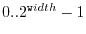Subsections

# Time evolution

Starting with version 0.9.1 libquantum provides an interface for simulating arbitrary quantum systems by numerically integrating the Schrödinger equation. The algorithm used is a fourth-order Runge-Kutta scheme [Press, 1992].

## quantum_rk4

 extern void quantum_rk4(quantum_reg *reg, double t, double dt, quantum_reg H(MAX_UNSIGNED, double));

This function performs a time-step of dt at time t, by calling the function H, which implements the Hamiltonian of the system. H is expected to return a quantum register containing a single row of the Hamiltonian. The expected row is specified by the first parameter to H. The second parameter is the time t, which allows you to solve time-dependent problems.

The best way to implement the Hamiltonian is as follows:

1. Determine the number of basis states needed for the current row
2. Create a quantum register with quantum_new_qureg_size
3. Fill in the values for the current row
4. Return the quantum register

Note that quantum_rk4 requires reg to be quantum register containing all possible basis states () in ascending order. Again, use quantum_new_qureg_size for initialization of the quantum register. See the example below for further details.

You can even use a limited set of quantum gates concurrently with function. However, using one of the following gates will result in undefined behavior:

• quantum_cnot
• quantum_toffoli
• quantum_unbounded_toffoli
• quantum_sigma_x
• quantum_sigma_y
• quantum_gate2

## quantum_rk4a

 extern double quantum_rk4a(quantum_reg *reg, double t, double *dt, double epsilon, quantum_reg H(MAX_UNSIGNED, double));

This function implements the same algorithm as quantum_rk4, but with an adaptive step size. All parameters correspond to quantum_rk4, except for epsilon, which specifies the desired accuracy. Returns the new step size.

## Example

This example shows an atom in a laser field, undergoing Rabi oscillations.

 ``` 1 2 3 4 5 6 7 8 9 10 11 12 13 14 15 16 17 18 19 20 21 22 23 24 25 26 27 28 29 30 31 32 33 34 35 36 37 38 39 40 41 42 43 44 45 46 ``` ```#include #include #include #define pi 3.14159265358979 const double DE = 1; const double g = 0.1; quantum_reg H(unsigned long long i, double t) { quantum_reg reg = quantum_new_qureg_size(2, 1); reg.node.state = i; reg.node.amplitude = i*DE; reg.node.state = 1^i; reg.node.amplitude = g*sin(DE*t); return reg; } int main() { double T = 2*pi/g; double dt = 0.01; double t; quantum_reg reg; reg = quantum_new_qureg_size(2, 1); reg.node.state = 0; reg.node.amplitude = 1; reg.node.state = 1; reg.node.amplitude = 0; for(t=0; t